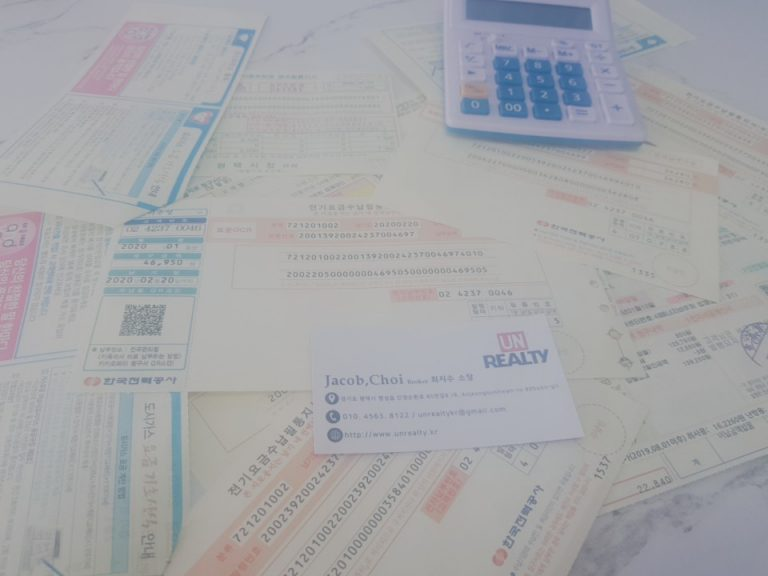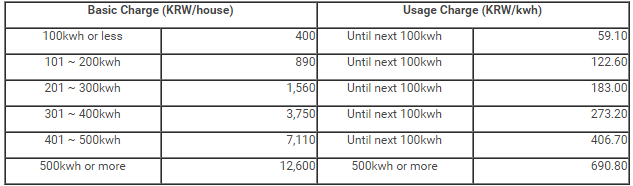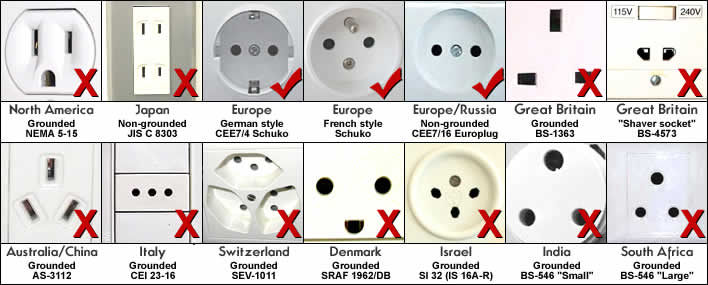USAG Humphreys UN Realty

# How to calculate your electric charges in KoreaIt is evident that extremely hot summer comes every year and this year it will be recorded the hottest temperature in all cities in South Korea hitting over 40 degree Celsius in some areas. As hot weather continues even at night, people are using air conditioners more and more at home which will definitely result in higher electricity bills.

Expatriates who are spending summer in Korea for the first time must know that electricity in Korea can be quite expensive and it sometimes can be doubled or tripled as your bill is calculated based on cumulative scheme; the rate of unit price for the electricity increases as the amount of electricity used. Previously we have seen quite a few expatriates who were surprised to see their electricity bill asking us about how the bill was calculated.To manage the usage of the electricity, it is essential to be aware of how the electric charges are calculated. Then, you should have an idea how much your electricity bill would show by checking and reading your electric meter.By using above chart, the calculation for usage of 490kwh/month is explained below.

100kwh = 100kwh = 59.10 * 100 = 5,910

200kwh = 100kwh = 122.60 * 100 = 12,260

300kwh = 100kwh = 183.00 * 100 = 18,300

400kwh = 100kwh = 273.20 * 100 = 27,320

490kwh = 90kwh = 406.70 * 90 = 36,603

Total usage charge = 5,910 + 12,260 + 18,300 + 27,320 + 36,603 = KRW 100,393

Total basic charge = 400 + 890 + 1,560 + 3,750 + 7,110 = KRW 13,710

Therefore, when 490kwh/month is used, the total charge is (KRW 13,710 + KRW 100,393) + 10% VAT = KRW 125,513.

The calculation for usage of 510kwh/month is explained below.

100kwh = 100kwh = 59.10 * 100 = 5,910

200kwh = 100kwh = 122.60 * 100 = 12,260

300kwh = 100kwh = 183.00 * 100 = 18,300

400kwh = 100kwh = 273.20 * 100 = 27,320

500kwh = 100kwh = 406.70 * 100 = 40,670

510kwh = 10kwh = 690.80 * 10 = 6,908

Total usage charge = 5,910 + 12,260 + 18,300 + 27,320 + 40,670 + 6,908 = KRW 111,368

Total basic charge = 400 + 890 + 1,560 + 3,750 + 7110 + 12,600 = KRW 26,310

Therefore, when 510kwh/month is used, the total charge is (KRW 26,310 + KRW 111,368) + 10% VAT = KRW 151,446.

Hopefully the calculation is not too complicated and it is useful for you to manage the electricity usage.